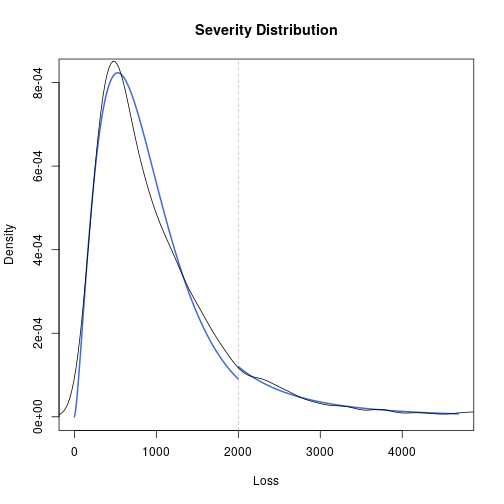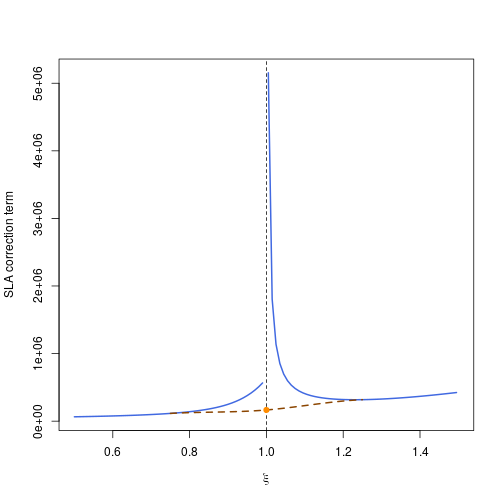# OpVaR: Modelling Operational (Value-at-)Risk in R

The package OpVaR is a toolkit of statistical methods for operational risk modeling. Anticipating a loss frequency/loss severity decomposition, it especially tackles the issues:

1. Flexible modeling of loss severity distributions (Spliced and Dynamic Mixture Models)
2. Total loss estimation considering bivariate dependencies between loss severities and frequencies (Copula Models, Monte-Carlo-Simuation)
3. Analytical closed form quantile approximations (Single Loss Approximations)

In the following, the functionality of OpVaR shall be briefly sketched using the hypothetical example data set lossdat. Load the package and this dataset by:

``````library(OpVaR)
data(lossdat)
``````

Key element of OpVaR is the opriskmodel structure. Inspired by the regulatory 8x7 business line/event type matrix, the opriskmodel structure is a list object where the single elements correspond e.g., to the cells of a business line/event type matrix. Each element comprises a loss frequency model, a loss severity model and if specified a dependency model between the frequencies and severities. Our lossdat dataset provides a minimal (2x2) example. As for the opriskmodel structure, the loss data needs to be stored in a list of cells with each cell comprising a data frame of loss severities and an integer time period assignment (e.g., years or quarters). With our example data set lossdat, a corresponding empty opriskmodel will comprise 4 cells/list elements and is initialised by:

``````opriskmodel=list()
for(i in 1:length(lossdat)){
opriskmodel[[i]]=list()
}
``````

## Fitting Loss Frequency Distributions

There are two options for modeling loss frequencies: the Poisson and Negative Binomial distribution. Loss frequency models are fitted using the fitFreqdist command and take into account the discrete time period classification in the data.

``````### Fit Frequency Distribution
opriskmodel[]\$freqdist=fitFreqdist(lossdat[],"pois")
opriskmodel[]\$freqdist=fitFreqdist(lossdat[],"pois")
opriskmodel[]\$freqdist=fitFreqdist(lossdat[],"nbinom")
opriskmodel[]\$freqdist=fitFreqdist(lossdat[],"nbinom")
``````

## Fitting Loss Severity Distributions

For loss severities three types of models are available:

1. “plain” - a single distribution (e.g., gamma, lognormal, Weibull, Tukey's g-h distribution)
2. “spliced” - a flexible combination of two distributions and a threshold (maximum likelihood estimation/Bayesian estimation as in Ergashev, Mittnik and Sekeris, 2013)
3. “mixing” - a dynamically weighted mixture model as in Frigessi et al., 2002.

In the following, we fit a plain Gamma distribution to the first cell and a plain Weibull distribution for the second cell of lossdat. For the third and forth cell we select spliced distributions with a fixed threshold and a threshold to be fitted.

``````### fit Severity Distributions
opriskmodel[]\$sevdist=fitPlain(lossdat[],"gamma")
opriskmodel[]\$sevdist=fitPlain(lossdat[],"weibull")
opriskmodel[]\$sevdist=fitSpliced(lossdat[],"gamma","gpd", method = "Fixed",thresh = 2000)
opriskmodel[]\$sevdist=fitSpliced(lossdat[],"gamma","gpd", method = "mindist")
``````

## Evaluating Model Fit

Goodness of fit tests are available for the continuous loss severity models (Kolmogorov-Smirnov test) as well as for the discrete loss frequency models (Chi-square test):

``````### Test Model Fit (Severities)
goftest(lossdat[],opriskmodel[]\$sevdist)
``````
``````## []
##
##  Two-sample Kolmogorov-Smirnov test
##
## data:  cell\$Loss and sim
## D = 0.21103, p-value < 2.2e-16
## alternative hypothesis: two-sided
``````
``````plot(opriskmodel[]\$sevdist)
lines(density(lossdat[]\$Loss))
````````````### Test Model Fit (Frequencies)
goftest(lossdat[],opriskmodel[]\$freqdist)
``````
``````##
##  Chi-squared test for given probabilities
##
## data:  frequencies
## X-squared = 28.243, df = 67, p-value = 1
``````

## Considering Bivariate Dependencies

If bivariate dependencies between loss frequencies and severities in the single cells shall be considered, copula models can be fitted using the fitDependency function. fitDependency is an interface to the VineCopula package and thus uses the VineCopula package encoding (0 = independence copula, 1 = Gaussian copula, 2 = t copula, 3 = Clayton copula, 4 = Gumbel copula, 5 = Frank copula, 6 = Joe copula, for details, see the BiCop function documentation in the VineCopula package). If independence is assumed, it can be either specified by the independence copula (0) or simply not be specified.

``````### Fit Dependency Model
opriskmodel[]\$dependency=fitDependency(lossdat[],6)
opriskmodel[]\$dependency=fitDependency(lossdat[],0)
opriskmodel[]\$dependency=fitDependency(lossdat[],4)
``````

## Total Loss Estimation by Monte Carlo Simulation

Given a correctly specified opriskmodel, total loss for the single cells can be estimated by Monte Carlo simulation (mcSim function). The simulation result should be stored, so that afterwards Value-at-Risk can be determined by the VaR function. Especially depending on complex dependency or loss severity models, the simulation can be time consuming.

``````### Monte Carlo Simulation
mc_out=mcSim(opriskmodel,100,verbose=FALSE)

### Value-at-Risk Calculation
VaR(mc_out,.95)
``````
``````##      95%      95%      95%      95%
## 67492.42 79093.60 46247.46 41995.81
``````

## Single Loss Approximation

A closed form approximation of Value-at-Risk can also be determined using a tail index based quantile approximation assuming independence of the loss severity and loss frequency. This procedure is numerically fast and can e.g., be used for validating Monte Carlo simualtion based risk figures.

``````### Benchmark: Value-at-Risk by Single Loss Approximation
sla(opriskmodel,.95)
``````
``````## OpRiskmodel - SLA:  95 %
## ----------------------------------------------
##           VaR              Interpolation
## Cell 1 :  55966.8990739074 FALSE
## Cell 2 :  64288.3331035468 FALSE
## Cell 3 :  61643.0792691836 FALSE
## Cell 4 :  52502.2602087465 FALSE
``````

In case that the spline based interpolation is needed, it can also be graphically shown, consider the following example:

``````opriskmodel2 = list()
opriskmodel2[] = list()
opriskmodel2[]\$sevdist = buildSplicedSevdist("lgamma", c(1.23, 0.012), "gpd", c(200, 716, 0.9), 2000, 0.8)
opriskmodel2[]\$freqdist = buildFreqdist("pois", 50)

#generate plot if interpolation was performed
sla(opriskmodel2, alpha = 0.95, plot = TRUE)
````````````## OpRiskmodel - SLA:  95 %
## ----------------------------------------------
##           VaR              Interpolation
## Cell 1 :  442902.585340625 TRUE
``````

## References

Degen, M. (2010): The calculation of minimum regulatory capital using single-loss approximations. The Journal of Operational Risk, 5(4), 3.

Dehler, K. (2017): Bayesianische Methoden im operationellen Risiko. Master's Thesis, Friedrich-Alexander-University Erlangen-Nuremberg.

Ergashev, B. et al. (2013): A Bayesian Approach to Extreme Value Estimation in Operational Risk Modeling. Journal of Operational Risk 8(4):55-81

Frigessi, A. et al. (2002): A Dynamic Mixture Model for Unsupervised Tail Estimation Without Threshold Selection. Extremes 5(3):219-235

Kuo, T. C. and Headrick, T. C. (2014): Simulating Univariate and Multivariate Tukey g-and-h Distributions Based on the Method of Percentiles. ISRN Probability and Statistics.

Pfaelzner, F. (2017): Einsatz von Tukey-type Verteilungen bei der Quantifizierung von operationellen Risiken. Master's Thesis, Friedrich-Alexander-University Erlangen-Nuremberg.

Reynkens, T. et al. (2017): Modelling Censored Losses Using Splicing: a global fit strategy with mixed Erlang and Extreme Value Distributions. Insurance: Mathematics and Economics 77:67-77

Tukey, J. W. (1960): The Practical Relationship between the Common Transformations of Counts of Amounts. Technical Report 36, Princeton University Statistical Techniques Research Group, Princeton.